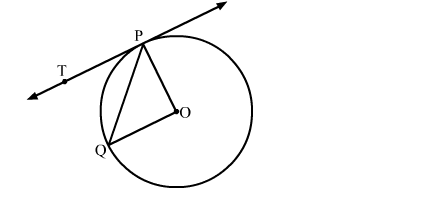# In the given figure, O is the centre of the circle, PQ is a chord and PT is the tangent at P.`
Question:

In the given figure, O is the centre of the circle, PQ is a chord and PT is the tangent at P. If ∠POQ = 70 , then ∠TPQ is equal to

(a) 35
(b) 45
(c) 55
(d) 70Solution:

We know that the radius and tangent are perperpendular at their point of contact
Since, OP = OQ
∵POQ is a isosceles right triangle
Now, In isoceles right triangle POQ
∠POQ + ∠OPQ + ∠OQP = 180            [Angle sum property of a triangle]
⇒  70 + 2∠OPQ = 180
⇒ ∠OPQ = 55
Now, ∠TPQ + ∠OPQ = 90
⇒ ∠TPQ = 35
Hence, the correct answer is option (a).